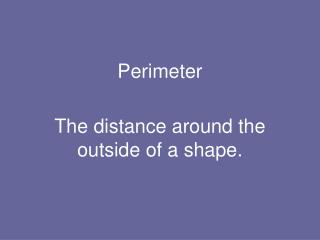DownloadDownload PresentationPerimeter

# Perimeter

Download Presentation## Perimeter

- - - - - - - - - - - - - - - - - - - - - - - - - - - E N D - - - - - - - - - - - - - - - - - - - - - - - - - - -
##### Presentation Transcript

1. Perimeter The distance around the outside of a shape.

2. To find the perimeter you need to measure the length of each side of the rectangle. Perimeter then add them together.

3. The distance around the outside of a circle. Distance across middle. Diameter Circumference Distance from middle to circumference. Radius

4. The distance around the outside of a rectangle. length Perimeter width width length Perimeter = (2 x L) + (2 x W)

5. Do you have to measure every side to find the perimeter of these shapes? Perimeter

6. Perimeter Word Problems

7. A regular pentagon has one side 25cm long.How long is the perimeter of this shape?

8. 2. A regular hexagon has a perimeter of 300cm.How long is the length of one side?

9. 2. A Hexagon has a perimeter of 300cm.How long is the length of one side?

10. 3. A square field has a perimeter of 1200m.How long is the length of each side of the field?One side of the field is hedged. What length of fencing is needed to fence in the other three sides?

11. What is the perimeter of a square of side 20cm?

12. If the perimeter of square is200cm. What is the length of one of the sides?

13. A rectangle has a perimeter of 68cm.It’s short side is 10cm. What is the length of the long side?